×

Search anything:

# Master the use of Set in Python

#### Software Engineering PythonReading time: 30 minutes | Coding time: 10 minutes

Set is a data structure which is a collection of objects. Python has an in-built support for set with common and useful operations like insert, delete, union, difference, subtraction and many more.

The sets module in Python provides classes for constructing and manipulating unordered collections of unique elements. We can perform all those kinds of operations on Sets in python as we can do in sets of maths. Some of them being:

2. Subtraction of elements of two sets,
3. Union,
4. Intersection,
5. Symmetric Difference.

The presence of an element in a set can be checked.

Both set and ImmutableSet derive from BaseSet which is an abstract class. Check if an object obj is a set or not:

``````isinstance(obj, BaseSet).
``````

Arrays, are data-structures where the elements are stored as ordered list, whereas the order of elements in a set is undefined.

Only immutable data types can be an element of a set:

1. a number
2. a string
3. a tuple

Mutable / changeable data types cannot be elements of the set.

• In particular, list cannot be an element of a set (but tuple can), and another set cannot be an element of a set. The requirement of immutability follows from the way how do computers represent sets in memory.

## Basic Operations on a single set

We will cover the following sub-topics:

• declaring and printing a set in Python
• adding elements, looping through elements and updating a set
• checking presence of an element, deleting an element
• set operations like union, intersection, subset and many more

### Declaring Set in Python

Declaration of sets is done by using the curly brackets '{','}'. Sets can have only immutable data types.

``````    A = {1, 2, 3}
print(A)
B = {}
print (B)
``````

Output:

``````{1, 2, 3}
{}
``````

### Printing elements of the Set

Print with the name of the set name will print all the elements of the set.

``````Sset = {"cow", "goat", "horse"}
print(Sset)
``````

Output:

``````{'horse', 'goat', 'cow'}
``````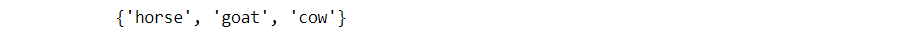### For loop on elements of a Set

Looping on elements of the the set, prints all the elements individually and not as a set.

``````sset = {"cow", "goat", "horse"}

for x in sset:
print(x)
``````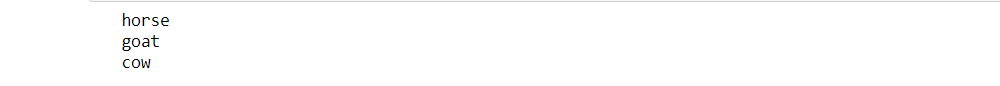### Return True on the presence of a particular element.

To check if an element is present in a set.

``````sset = {"cow", "goat", "horse"}

print("horse" in sset)
``````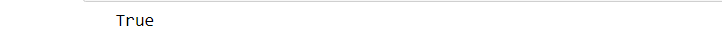### Add elements to the Set

``````sset = {"cow", "goat", "horse"}

print(sset)
``````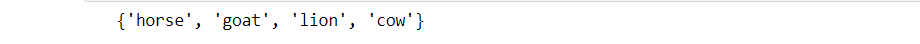### Update elements of a Set

To update the set.

``````sset = {"cow", "goat", "horse"}
sset.update(["giraffe", "turtle", "monkey"])

print(sset)
``````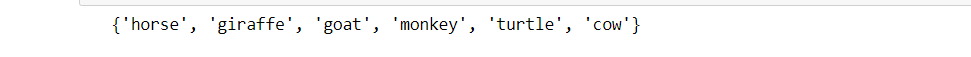## Question

#### set = {"Hello","Hola"}, set.update(["Bye","later"]). What is the output?

{'Hello','Hola'}
{'Bye','Later'}
{'Hello','Later'}
{'Bye','Hola'}
Great Job!

### Printing length of a Set

We can print the length i.e the number of elements present in a Set.

``````sset = {"cow", "goat", "horse"}
print(len(sset))
``````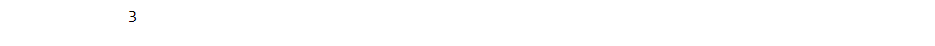### Removing a particular element of a Set

We can particularly remove an element of a Set.

``````sset = {"cow", "goat", "horse"}

sset.remove("goat")

print(sset)
``````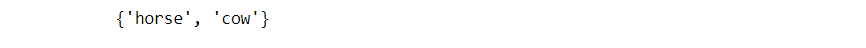### Discarding an element of a Set

``````sset = {"cow", "goat", "horse"}

print(sset)
``````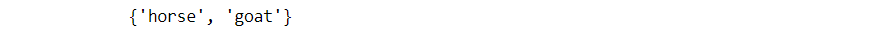### Popping first element of a Set

This example shows the popping of front element from a Set.

``````sset = {"cow", "goat", "horse"}

x = sset.pop()

print(x)

print(sset)
``````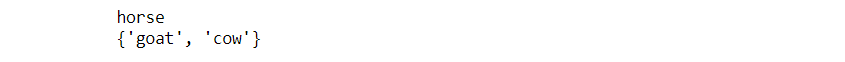### Clearing all elements of a Set

To clear all the elements of a Set.

``````sset = {"cow", "goat", "horse"}

sset.clear()

print(sset)
``````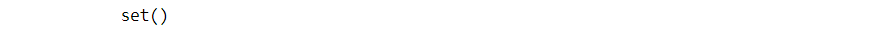### Deleting a Set

If we delete a Set. We get the following error as shown in output.

``````sset = {"cow", "goat", "horse"}

del sset

print(sset)
``````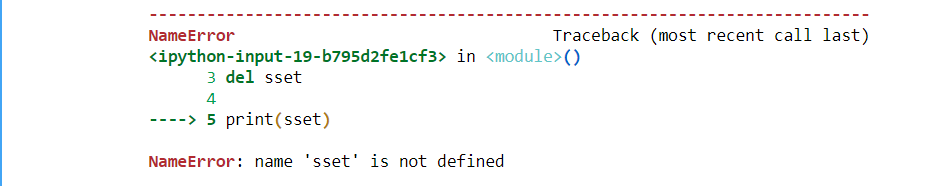Syntax Error
Error
{'Hello','Hola'}
{'Bye','later'}
Great Job!

# Operations on Sets

### Union of two Sets

If we want elements of both the Sets. We use the '|' or operator to get the output as shown.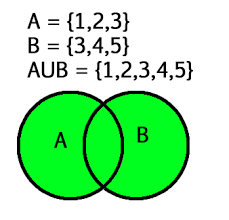``````set1 = {1,2,3}
set2 = {4,5,6}
set3 = set1 | set2
print(set3)
``````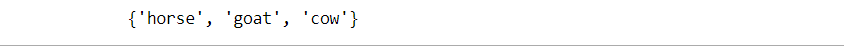### Intersection of two Sets

If intersection of the elements of two Sets, is required. Then we use the '&' and operator, and the result can be seen in the output.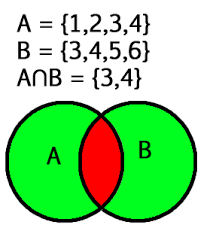``````set1 = {1,2,3}
set2 = {3,4,1}
set3 = set1 & set2
print(set3)
``````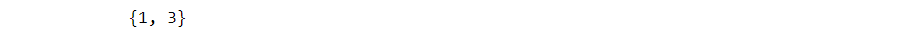## Question

Syntax Error
Error
set()
{1,2,3,4}
Great Job!

### Check Subset (issubset)

We can check if a set is a subset of another Set. By using 'issubset' operator.

``````set3 = {1,2,3}
set4 = {1,3}
if set4 < set3: # set4.issubset(set3)
print("Set4 is subset of Set3")
``````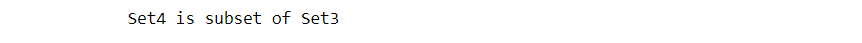### Difference between elements of two sets.

``````set3 = {1,2,3}
set4 = {1,3}
set5 = set3 - set4
print("Elements in Set3 and not in Set4: Set5 = ", set5)
``````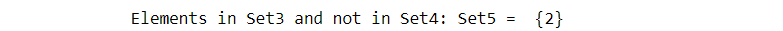### isDisjoint

Using this element we can check, if two sets are disjoint or they have something in common.

``````set4 = {1,2,3}
set5 = {4,5}
if set4.isdisjoint(set5):
print("Set4 and Set5 have nothing in common\n")
``````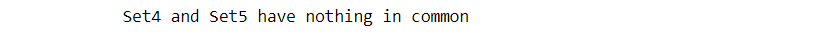Yes
No
Great Job!